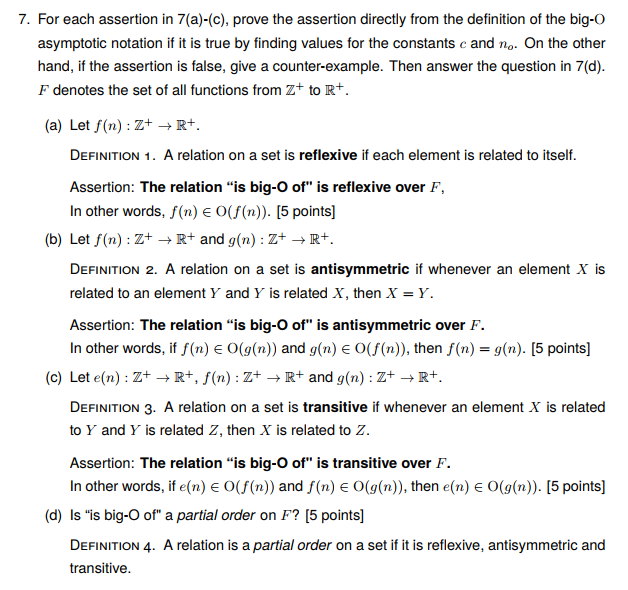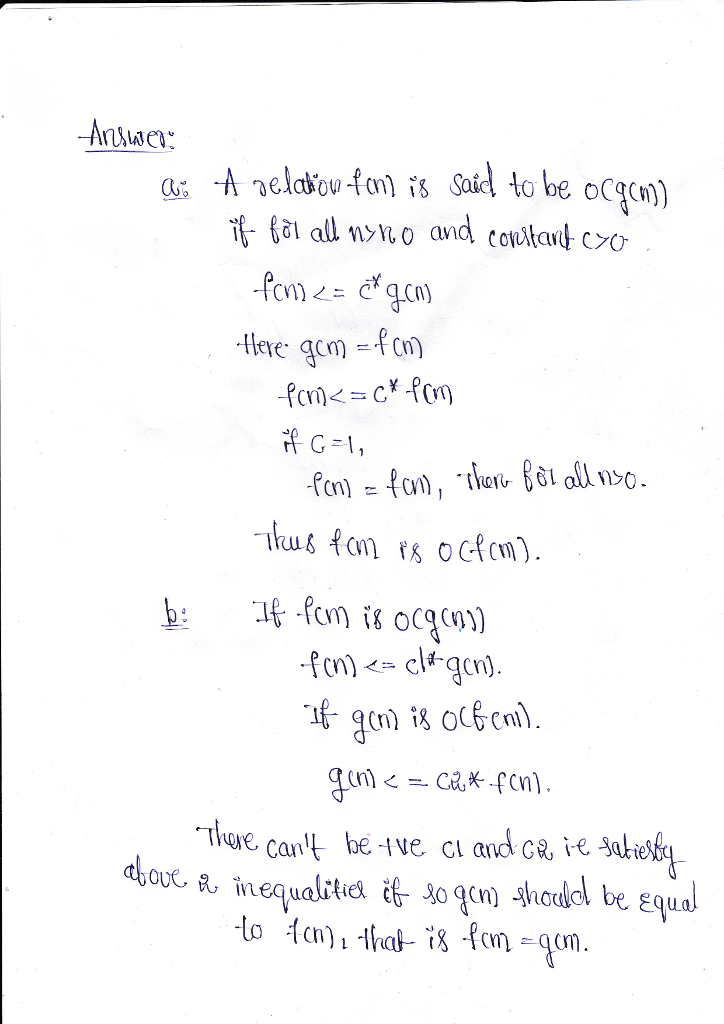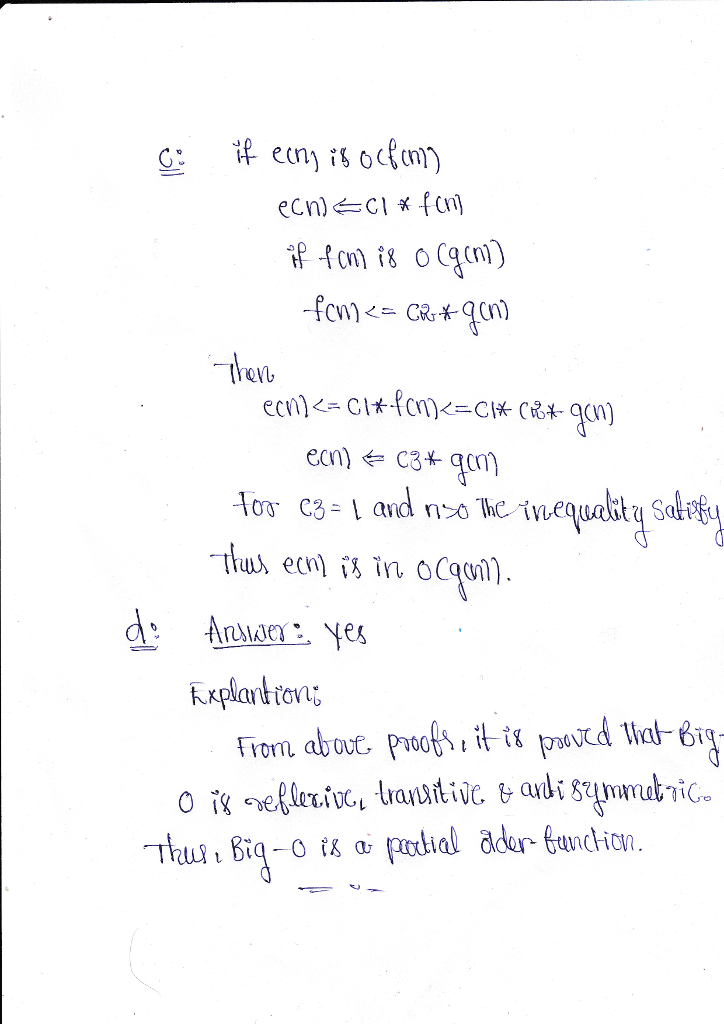# Homework Solution: For each assertion in 7(a)-(c), prove the assertion directly from the definition of the big-O asymptotic n…For each assertion in 7(a)-(c), prove the assertion directly from the definition of the big-O asymptotic notation if it is true by finding values for the constants c and n_0. On the other hand, if the assertion is false, give a counter-example. Then answer the question in 7(d) F denotes the set of all functions from Z^+ to R^+. (a) Let f(n): Z^+ rightarrow R^+. DEFINITION 1. A relation on a set is reflexive if each element is related to itself. Assertion: The relation "is big-O of" is reflexive over F, In other words, f(n) elementof O(f(n)). (b) Let f(n): Z^+ rightarrow R^+ and g(n): Z^+ rightarrow R^+. DEFINITION 2. A relation on a set is antisymmetric if whenever an element X is related to an element Y and Y is related X, then X = Y. Assertion: The relation "is big-O of" is antisymmetric over F. In other words, if f(n) elementof O(g(n))and g(n) elementof O(f(n)), then f(n) = g(n). (c) Let e(n): Z^+ rightarrow R^+, f(n): Z^+ rightarrow R^+ and g(n): Z^+ rightarrow R^+. DEFINITION 3. A relation on a set is transitive if whenever an element X is related to Y and Y is related Z, then X is related to Z. Assertion: The relation "is big-O of" is transitive over F. In other words, if e(n) elementof O(f(n)) and f(n) elementof O(g(n)), then e(n) elementof O(g(n)). (d) Is "is big-O of" a partial order on F? DEFINITION 4. A relation is a partial order on a set if it is reflexive, antisymmetric and transitive.